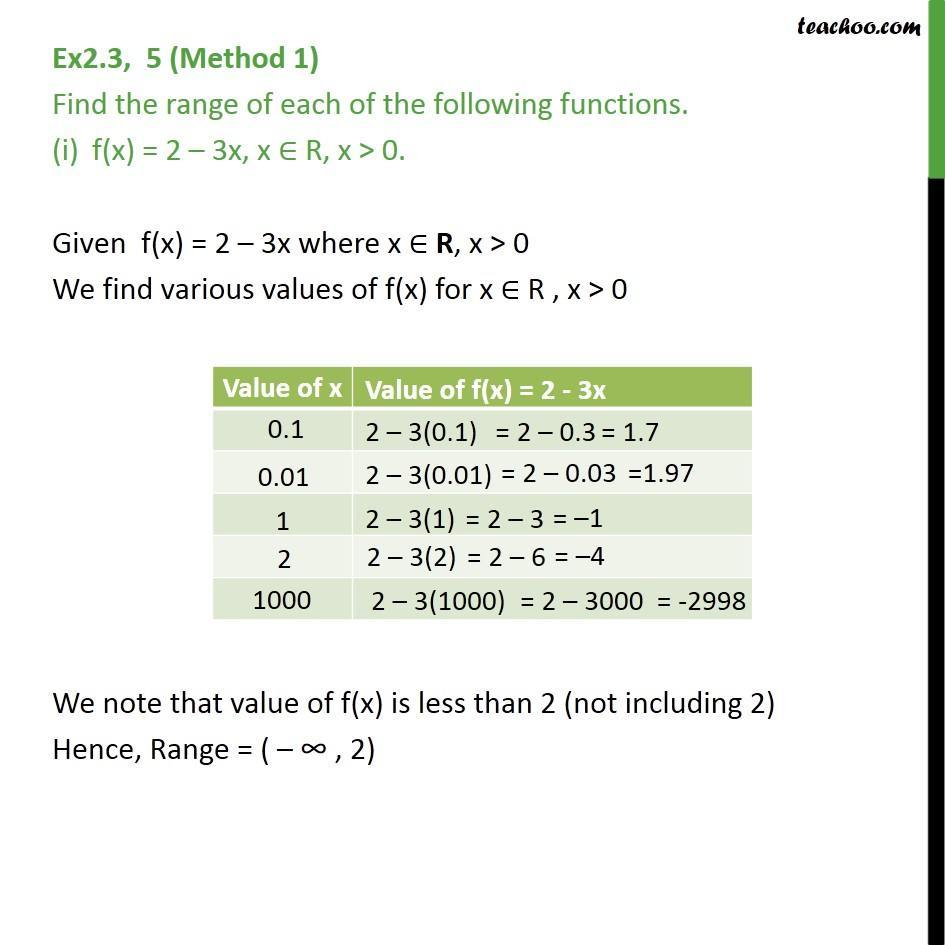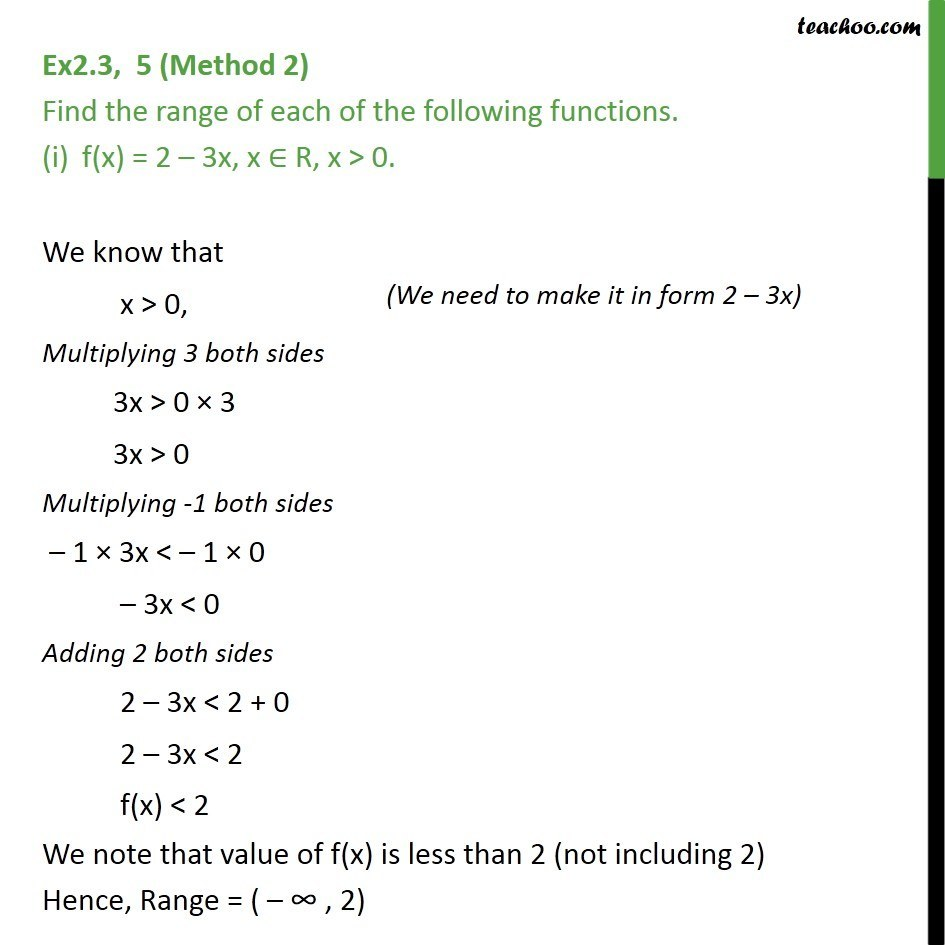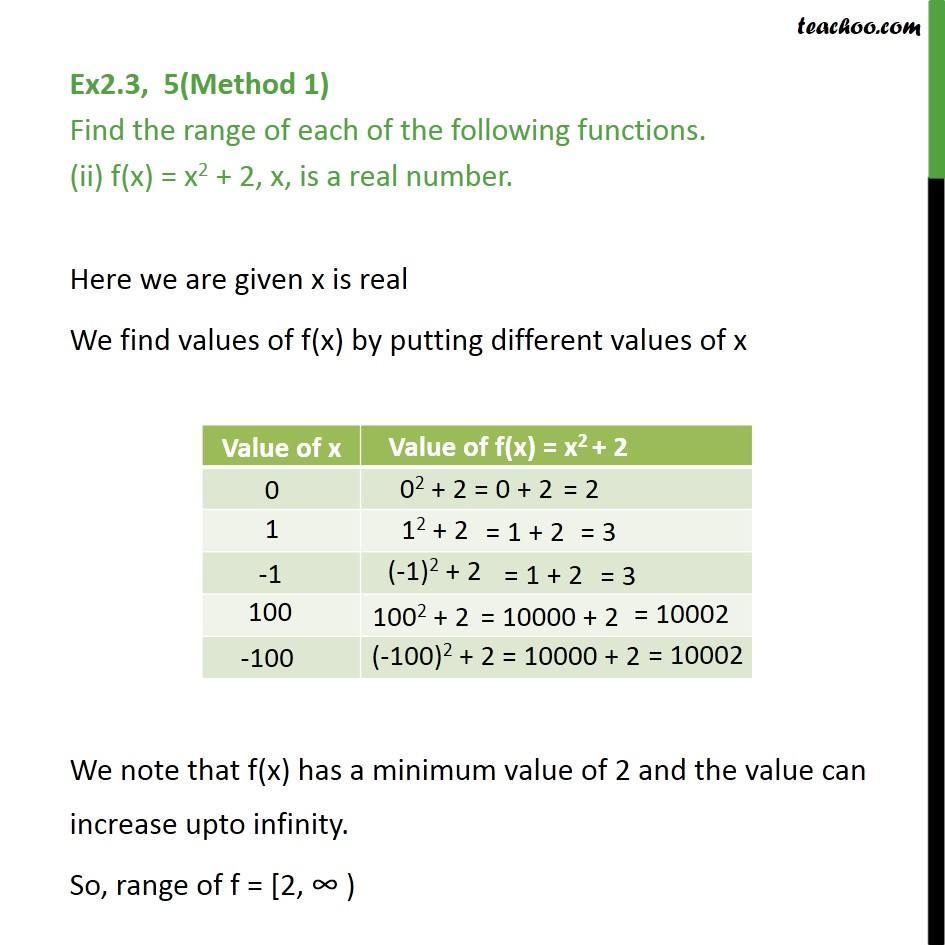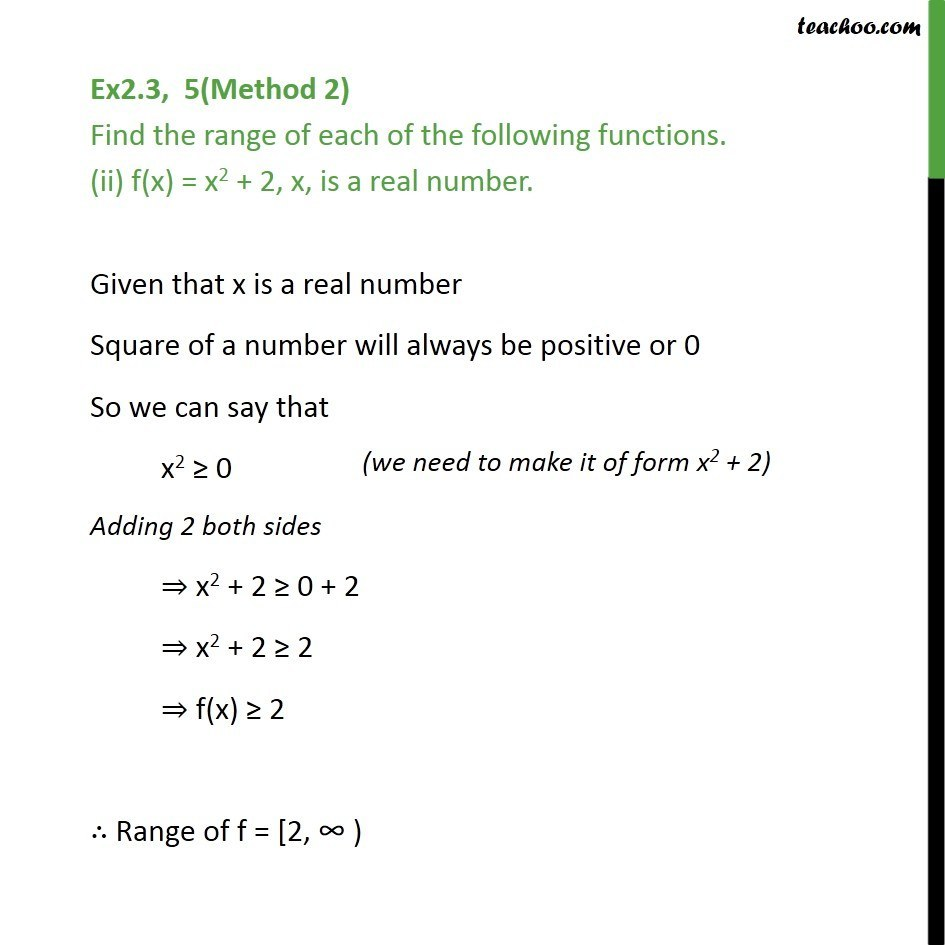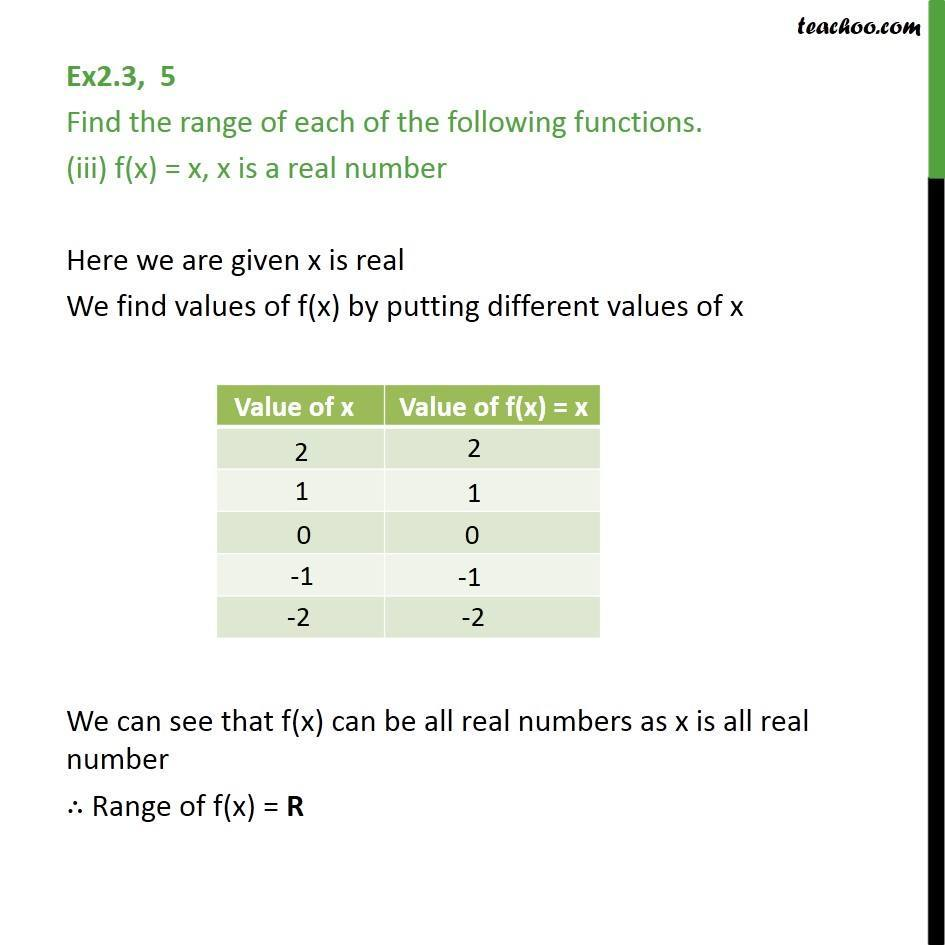1. Chapter 2 Class 11 Relations and Functions
2. Serial order wise
3. Ex 2.3

Transcript

Ex2.3, 5 (Method 1) Find the range of each of the following functions. f(x) = 2 – 3x, x ∈ R, x > 0. Given f(x) = 2 – 3x where x ∈ R, x > 0 We find various values of f(x) for x ∈ R , x > 0 We note that value of f(x) is less than 2 (not including 2) Hence, Range = ( – ∞ , 2) Ex2.3, 5 (Method 2) Find the range of each of the following functions. f(x) = 2 – 3x, x ∈ R, x > 0. We know that x > 0, Multiplying 3 both sides 3x > 0 × 3 3x > 0 Multiplying -1 both sides – 1 × 3x < – 1 × 0 – 3x < 0 Adding 2 both sides 2 – 3x < 2 + 0 2 – 3x < 2 f(x) < 2 We note that value of f(x) is less than 2 (not including 2) Hence, Range = ( – ∞ , 2) Ex2.3, 5(Method 1) Find the range of each of the following functions. (ii) f(x) = x2 + 2, x, is a real number. Here we are given x is real We find values of f(x) by putting different values of x We note that f(x) has a minimum value of 2 and the value can increase upto infinity. So, range of f = [2, ∞ ) Ex2.3, 5(Method 2) Find the range of each of the following functions. (ii) f(x) = x2 + 2, x, is a real number. Given that x is a real number Square of a number will always be positive or 0 So we can say that x2 ≥ 0 Adding 2 both sides ⇒ x2 + 2 ≥ 0 + 2 ⇒ x2 + 2 ≥ 2 ⇒ f(x) ≥ 2 ∴ Range of f = [2, ∞ ) Ex2.3, 5 Find the range of each of the following functions. (iii) f(x) = x, x is a real number Here we are given x is real We find values of f(x) by putting different values of x We can see that f(x) can be all real numbers as x is all real number ∴ Range of f(x) = R

Ex 2.3